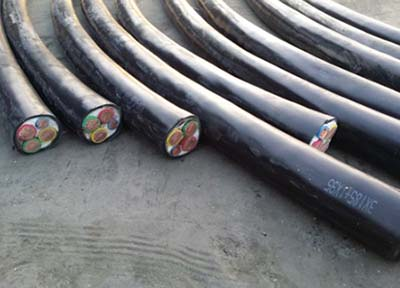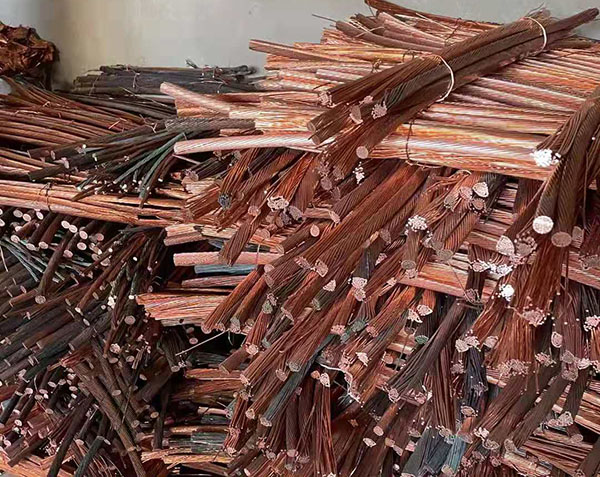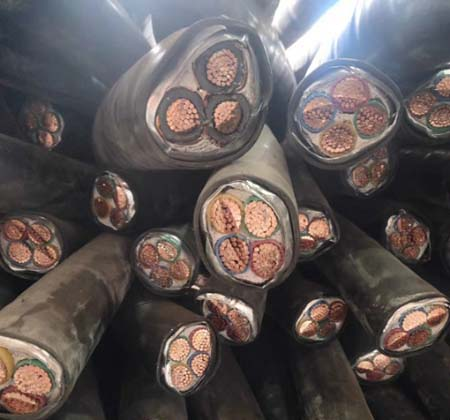# 废旧铜电缆回收时含铜量如何计算,电缆回收公司电话135216633531、每千米导体重量：(Kg/Km)=d2×0.7854×G×N×K1×K2×C
d=铜线径 ? ?G=铜比重 ? ?N=条数 ? ?K1=铜线绞入率 ? ?K2=芯线绞入率 ? ?C=绝缘芯线根数

2、每千米绝缘重量：(Kg/Km)=(D2-d2)×0.7854×G×C×K2
D=绝缘外径 ? ?d=导体外径 ? ?G=绝缘比重 ? ?K2=芯线绞入率 ? ?C=绝缘芯线根数3、每千米外被重量：(Kg/Km)=(D12-D2)×0.7854×G
D1=完成外径 ? ?D=上过程外径 ? ?G=绝缘比重

4、每千米包带重量：(Kg/Km)=D2×0.7854×t×G×Z
D=上过程外径 ? ?t=包带厚度 ? ?G=包带比重 ? ?Z=重叠率(1/4Lap=1.25)

5、每千米缠绕重量：(Kg/Km)=d2×0.7854×G×N×Z
d=铜线径 ? ?N=条数 ? ?G=比重 ? ?Z=绞入率

6、每千米编织重量：(Kg/Km)=d2×0.7854×T×N×G/cosθ
θ=atan(2×3.1416×(D+d×2))×目数/25.4/T
d=编织铜线径 ? ?T=锭数 ? ?N=每锭条数 ? ?G=铜比重7、每千米铜电缆重量(Kg/Km)=铜导体重量+绝缘体重量+外被重量+包带重量+缠绕物重量+编织物重量

### 联系方式

13521663353•#### 在线客服

##### 在线咨询
•#### 联系电话

##### 热线电话

13521663353

•#### 添加微信

##### 添加微信获取报价•